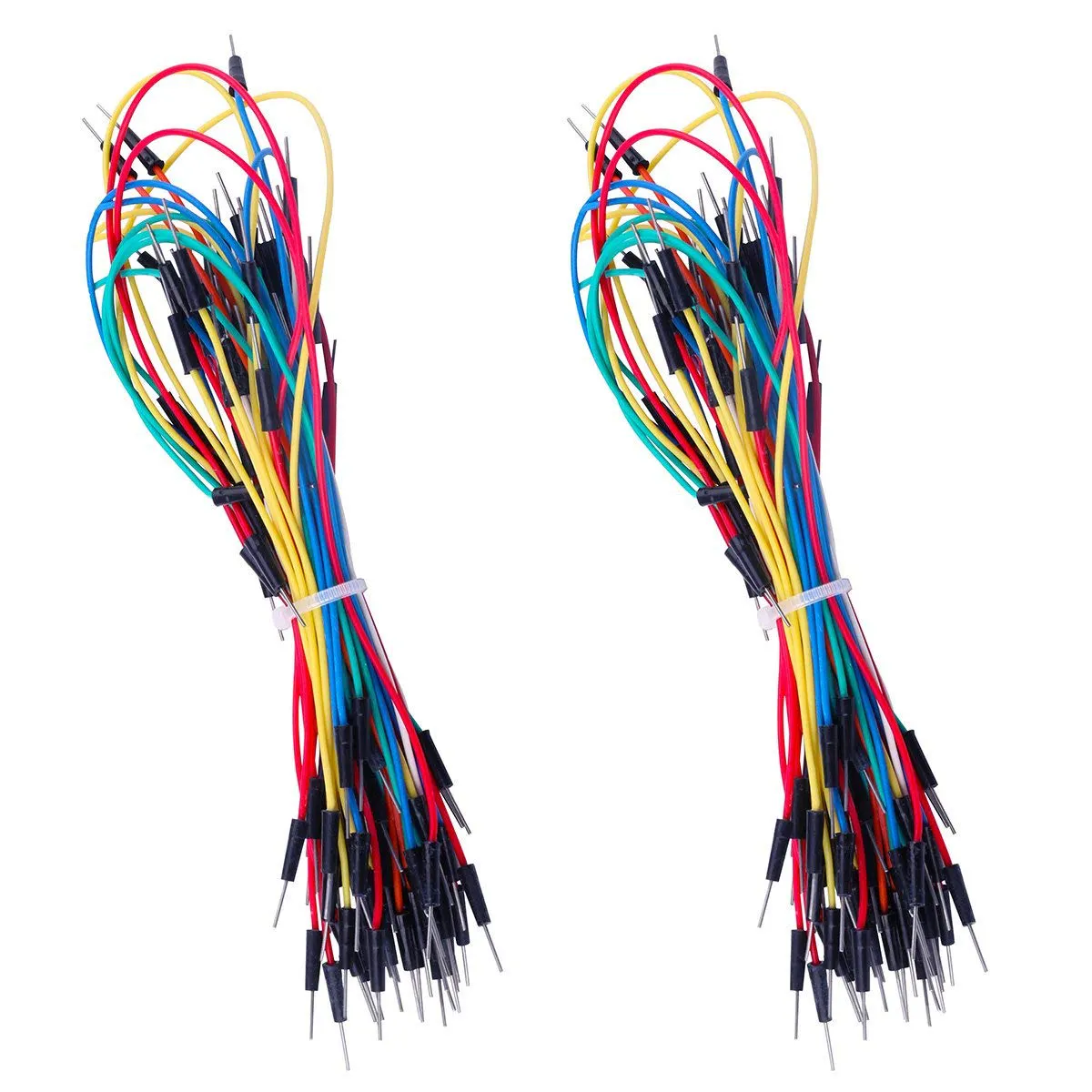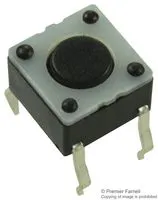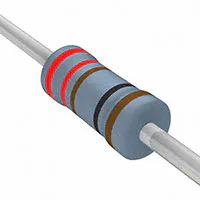Project tutorial# Entangled Qubits Simulator with Arduino © LGPL

It is a visual representation of qubits entangled or not. Qubits are simulated with an Arduino firmware, by a biased randomness & collapsed.

• 1,422 views
• 5 respects

## Components and supplies

 ELK-COGITI Material Para Curso COGITI ELK-COGITI Material Para Curso COGITI This is material for a course of collegiated engineers in Galicia (Spain) mainly. The kit cotaines a lot of components, not all necessary. In case someone prefers to buy components separately, I suggest the ones bellow
×1LED (generic)
×3ELEGOO 130pcs Solderless Flexible Breadboard Jumper Wires 4 Different Lengths Male To Male
×1Tactile Switch, Top Actuated To stop/resume the firmaware qubits_six_OR_nov10_2020.ino
×1Resistor 221 ohm 220-390 ohm
×3Arduino UNO
×1×1

## Apps and online services

### Entanglement

I was curious about quantum computing and wanted to see what was all about. I learned some basics. I realize that superposition was similar to a biased randomness and that entangled can be represented with a formula which relates two qubits :

``correlated ? (bQubit = aQubit = (aCollapse ? collapse(aPercentOne) : collapse(bPercentOne))) : (correlated = 0); //qubits entangled``

Qubits are like bits but can be at the same time a percentage of |0> and a percentage (until 100% in total) of |1>. If they are entangled, the one that collapses first, "drags" the other to the same state or the contrary, depending on how are they correlated. I use the words correlated and entanglement indistinctly.

### Superposition

For simulated superposition we need biased aleatoriness, For creating true randomness we need a "seed" based on analog pin A0 or any other.

``randomSeed(analogRead(A0));``

If we created a randomness which starts and finish different of 0 and 200 we biased the behavior of qubits. That provides overlap very similar to the real one.

``//Ex. percent of be a one: 25%bool collapse(int percentOne) {int percentZero = 100 - percentOne;return random(100 - percentZero, 100 + percentOne) > 100 ? 1 : 0;}``

### qubit_entangled.ino firmware

For representing the quantum bits, you can use LEDs.

Once loaded the first program, qubit_entangled.ino, It is important to open the Serial Monitor and answer the questions asked:

• Percentages of being a one of both qubits
• If they are entangled
• Which qubit collapse first.

Before, you can mount the circuit:

It not necessary to use the button and the third LED for this example of code.

### qubits_six_OR_nov10_2020 firmware

This second code what does is a operation with two pair of three qubits. Although we do not use instructions of quantum computing, we use AND an OR operations that have an equivalence in other similar in quantum computing.

This firmware requires the three LEDs-Qubits and the button to stop/resume the program

### Conclusion

With each firmware, we can see the behavior of qubits depending on the percentages of being |1> and |0> of each one. LEDs also provide a better comprehension. And Serial Monitor helps. And I think that the set simulates very well qubits using only Arduino.

I have not pretend being exact but creative in all case.

### Possible Bettering

In my opinion we could use a joystick for change the percentage of being |0> and consequently of being |1> of two qubits in real time, so learn speedily how affects the final collapses. A joystick would no more that two potentiometers, one dedicated for each qubit.

## Code

##### qubit_entangled.inoArduino
The button does not work with this firmware. It is not necessary
```/*qbits are in superposition and can be entangled (correlated)
* We substitute superposition by biased randomness and entanglement by a depending equation
*/
int aPercentOne, bPercentOne;
int aQubit, bQubit; //qubits
bool aCollapse, bCollapse;
String collapsed;
String entangled;
int correlated; //0: not correlated, 1: correlated, 2 error;
bool error;
#define doNothing 2
#define LEDq2 6 //they represent the state of qubits
#define LEDq1 7

void setup() {
Serial.begin(115200);

Serial.print("\nProbabillity of first qubit of being a 1.  0%-100%:");
while (!Serial.available()) {};
printQubit(aPercentOne);

Serial.print("Probablillity of second qubit of being a 1.  0%-100%:");
while (!Serial.available()) {};
printQubit(bPercentOne);
//qubits entangled
do {
Serial.print("Are those qubits entangled? Y/N:");
while (!Serial.available()) {};
entangled.trim();
correlated = (entangled == "Y" || entangled == "y") ? 1 : ((entangled == "N" || entangled == "n") ? 0 : doNothing) ;
} while (correlated == doNothing);
Serial.print(" ");
correlated ? Serial.println("Qubits ENTANGLED") : Serial.println("not entangled");
if (correlated) {
Serial.print("Both qubits are entangled. Which qubit collapses firts? A/B:");
while (!Serial.available()) {};
collapsed.trim(); //do not forget
collapsed.toLowerCase();
Serial.print(" ");
Serial.println(collapsed);
aCollapse = collapsed == "a" ? 1 : 0;
bCollapse = collapsed == "b" ? 1 : 0;
}
}

void loop() {
//the following are the most interested formulas of the present firmware
//collapse qubits and show with LEDs
//entangled, use the same formula
correlated ? (bQubit = aQubit = (aCollapse ? collapse(aPercentOne) : collapse(bPercentOne))) : (correlated = 0); //qubits entangled
//not entangled, use different formulas
!correlated ? aQubit = collapse(aPercentOne) : doNothing; //if not entangled collapse
!correlated ? bQubit = collapse(bPercentOne) : doNothing; //if not entangled collapse
//show by Serial Monitor
Serial.print("ba = ");
Serial.print(bQubit); digitalWrite(LEDq2, bQubit); //present the qubits by Serial Monitor and visually
//show by LEDs
Serial.println(aQubit); digitalWrite(LEDq1, aQubit);
delay(1000);
}
//Ex. percent of be a one: 25%
bool collapse(int percentOne) {
int percentZero = 100 - percentOne;
return random(100 - percentZero, 100 + percentOne) > 100 ? 1 : 0;
}

void printQubit(int percentOne){

Serial.println();
//when qubits are |0> or |1>
if (percentOne == 0 || percentOne == 100) {
percentOne == 100 ? Serial.println("|1>") : doNothing;
percentOne == 0 ? Serial.println("|0>") : doNothing;
}
else {
Serial.print("((√");
Serial.print(100-percentOne); //print percentages of first qubit
Serial.print(")/10)|0> + ");
Serial.print("((√");
Serial.print(percentOne);
Serial.println(")/10)|1>\n");
}
}
```
##### qubits_six_OR_nov10_2020Arduino
Makes operation with to groups of three qubits in superposition. Instead of quantum operation are use and OR or an AND operation, that can be choose inside the firmware. The button is used to stop/resume the firmware
```/* OR (or AND) of six qubits (in subsets of 3,)without entanglemet, and that then they collapse
*  Superposition is substituited by a biased random
* Author: Jose Romaní, from Vigo (Galicia, Spain)
*
* for example:
* q0 12% of being 1, 88% of being 0
* q1 30% of being 1, 70% of being 0
* q2 75% of being 1, 25% of being 0
*
* q3 50% of being 1, 50% of being 0
* q4 25% of being 1, 75% of being 0
* q5 60% of being 1, 40% of being 0
*
* find out the probabilities of every combination
* test 100 or 1000 measures to see what happens
* q52 q41 q30 measures=1000(OR)  measures=1000 (AND)
* 0   0    0      2.7%                    44%
* 0   0    1      1.6%                   2.0%
* 0   1    0      0.9%                   2.9%
* 0   1    1      1.5%                     0%
* 1   0    0     27.6%                  46.1%
* 1   0    1     20.4%                     2%
* 1   1    0     25.6%                     3%
* 1   1    1     19.7%                     0%
*/

const int measures = 100; //number of loop repetitions. 100 Or 1000 for example.
int q0, q1, q2, q3, q4, q5; //Qubits
int q52, q41, q30; //q52 is the result of an OR between q5 and q2. It is only a notation of mine
int q52q41q30; //qubits resulting of the OR
int percentOneQ0, percentOneQ1, percentOneQ2; //percentages
int percentOneQ3, percentOneQ4, percentOneQ5; //percentages
int j = 0;
//define a operation to apply
#define OPERATION_OR_bitwise |
#define OPERATION_AND_bitwise &
#define OPERATION OPERATION_OR_bitwise //for an AND substitute by OPERATION_AND_bit &
#define doNothing 2
int number000;
int number001;
int number010;
int number011;
int number100;
int number101;
int number110;
int number111;
#define LEDq52 6
#define LEDq41 7
#define LEDq30 8
#define BUTTON 2  //stop/resume the firmware
volatile bool state = false;
void setup() {
pinMode(LEDq52, OUTPUT);
pinMode(LEDq41, OUTPUT);
pinMode(LEDq30, OUTPUT);
digitalWrite(LEDq52, LOW); //LEDs OFF
digitalWrite(LEDq41, LOW);
digitalWrite(LEDq30, LOW);
pinMode(BUTTON, INPUT_PULLUP); //stops/resume the firmware

Serial.begin(115200);
//Stop until a key is pressed
Serial.println("Introduce any text to start and pulse key \"return\"\n");
while(!Serial.available()){}
delay(500);
percentOneQ0 = 12; //12% of being 1
percentOneQ1 = 30;
percentOneQ2 = 75;
percentOneQ3 = 50; //50% of being 1
percentOneQ4 = 25;
percentOneQ5 = 60;

attachInterrupt(digitalPinToInterrupt(BUTTON), changeState, RISING);
state = false;//put after attachInterrupt, if not, it stops the firmware
Serial.println("q52q41q130:");
}

void loop() {
if (state){
//switch off LEDs
digitalWrite(LEDq30, LOW);
digitalWrite(LEDq41, LOW);
digitalWrite(LEDq52, LOW);
delay(100);
return;
}
//collapse
q0 = (random(percentOneQ0, 100 + percentOneQ0) > 100) ? 1 : 0; //qubit collapsed
q1 = (random(percentOneQ1, 100 + percentOneQ1) > 100) ? 1 : 0;
q2 = (random(percentOneQ2, 100 + percentOneQ2) > 100) ? 1 : 0;

q3 = (random(percentOneQ0, 100 + percentOneQ3) > 100) ? 1 : 0; //qubit collapsed
q4 = (random(percentOneQ1, 100 + percentOneQ4) > 100) ? 1 : 0;
q5 = (random(percentOneQ2, 100 + percentOneQ5) > 100) ? 1 : 0;

//OR between the two groups of qubits
//Intention is that they do not collapse until now, with the randoms and percentages
//A biased random is similar to a superposition
//q30 = q3 OPERATION q0
//q41 = q4 OPERATION q1
//q52 = q5 OPERATION q2
q30 = ((random(percentOneQ0, 100 + percentOneQ3) > 100) ? 1 : 0) OPERATION ((random(percentOneQ0, 100 + percentOneQ0) > 100) ? 1 : 0);
q41 = ((random(percentOneQ1, 100 + percentOneQ4) > 100) ? 1 : 0) OPERATION ((random(percentOneQ1, 100 + percentOneQ1) > 100) ? 1 : 0);
q52 = ((random(percentOneQ2, 100 + percentOneQ5) > 100) ? 1 : 0) OPERATION ((random(percentOneQ2, 100 + percentOneQ2) > 100) ? 1 : 0);
//final state
q52q41q30 = (q30 | (q41 << 1)) | (q52 << 2); //all qubits together;

//Show state with LED
digitalWrite(LEDq30, q30);
digitalWrite(LEDq41, q41);
digitalWrite(LEDq52, q52);

printQubits();
printRepetitions();

j++;
Serial.println(j); //number of loops
if (j >= measures){ //repeat n times
digitalWrite(LEDq52, LOW); //LEDs OFF
digitalWrite(LEDq41, LOW);
digitalWrite(LEDq30, LOW);
while (1); //stop the process
}
}

void printQubits() {

//print on serial monitor all the three collapsed qubits
Serial.print(q52q41q30 >> 2);
Serial.print((q52q41q30 & B010) >> 1);
Serial.println(q52q41q30 & B001);
delay(1000);
}

void printRepetitions(){ //counts number of collapsed qubits repetitions
Serial.print("000: ");
Serial.print(number000);
Serial.print("   ");
Serial.print("001: ");
Serial.print(number001);
Serial.print("   ");
Serial.print("010: ");
Serial.print(number010);
Serial.print("   ");
Serial.print("011: ");
Serial.print(number011);
Serial.print("   ");
Serial.print("100: ");
Serial.print(number100);
Serial.print("   ");
Serial.print("101: ");
Serial.print(number101);
Serial.print("   ");
Serial.print("110: ");
Serial.print(number110);
Serial.print("   ");
Serial.print("111: ");
Serial.print(number111);
Serial.println();
}

q52q41q30 == B000 ? number000++ : doNothing;
q52q41q30 == B001 ? number001++ : doNothing;
q52q41q30 == B010 ? number010++ : doNothing;
q52q41q30 == B011 ? number011++ : doNothing;
q52q41q30 == B100 ? number100++ : doNothing;
q52q41q30 == B101 ? number101++ : doNothing;
q52q41q30 == B110 ? number110++ : doNothing;
q52q41q30 == B111 ? number111++ : doNothing;
}

void changeState(){
state = !state;
}
```

## Schematics

qubits_led_button_-_copy_uJhOxr8KCS.fzz

#### Published on

November 16, 2020

#### Members who respect this project

See similar projects
you might like

#### Arduino Traffic Light Simulator

Project tutorial by Zachary Haslam

• 77,571 views
• 100 respects

#### Light Sequence Creator

Project tutorial by Reid Paulhus

• 20,532 views
• 42 respects

Project tutorial by Super Kid

• 8,007 views
• 21 respects

#### Arduino 4WD RC Car

Project tutorial by Andriy Baranov

• 58,743 views
• 113 respects

#### Serial Arduino (Control the PWM)

Project tutorial by Super Kid

• 8,786 views
• 1 comment
• 5 respects

#### Interactive LED Name

Project tutorial by Pedro Mendez and Daniel Jablonski

• 4,713 views